A.3 WKB Formulas for Different Shapes of Energy Barriers

In the classical forbidden region, the shape of the wavefunction is dominated by the exponential term in equation (A.22).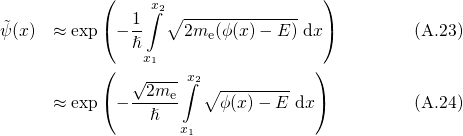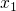and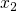stand for the classical turning point at the semiconductor-dielectric interface and the position of the trap, respectively. Supposing that only a negligible amount of charges is located in the dielectric, the potential energy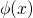can be expressed as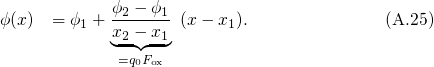For a trapezoidal barrier (see Fig. A.2),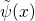simplifies to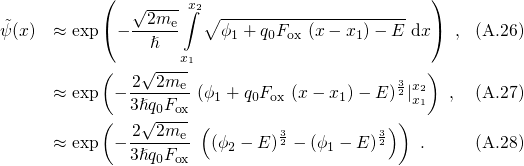If tunneling occurs through a triangular barrier (see Fig. A.2), the classically forbidden region extends to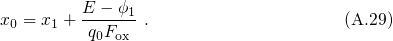For negative electric fields (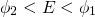), one obtains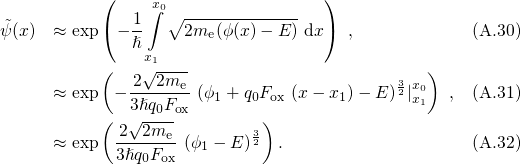while positive electric fields (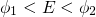) results in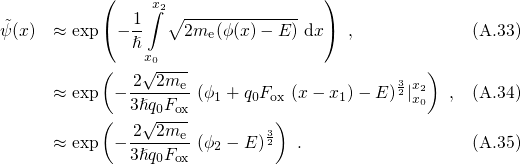The these two cases are commonly known as the Fowler-Nordheim formulas . For a rectangular barrier with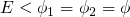, the integral in equation (A.23) simplifies to a multiplication.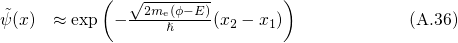Figure A.2: Schematic representation of a trapezoidal (left) and a triangular barrier for a negative (middle) and a positive (right) gate bias.displays the shape of the potential energy and takes the values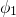and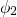for the semiconductor-dielectric interface at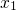and the trap at. For the case of triangular barriers, the classical forbidden region is decreased to the point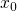.